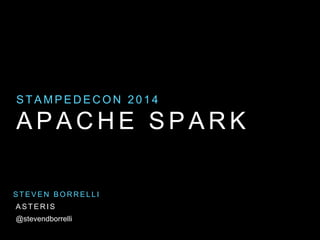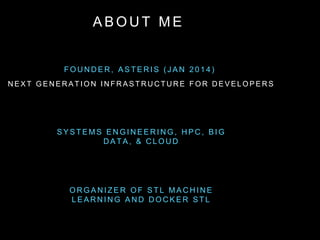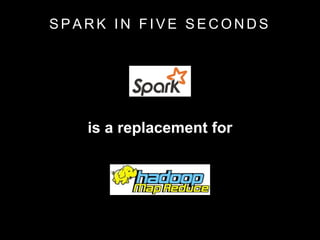Successfully reported this slideshow.

# Apache Spark: the next big thing? - StampedeCon 2014×

# Apache Spark: the next big thing? - StampedeCon 2014

Apache Spark: the next big thing? - StampedeCon 2014
Steven Borrelli
It’s been called the leading candidate to replace Hadoop MapReduce. Apache Spark uses fast in-memory processing and a simpler programming model to speed up analytics and has become one of the hottest technologies in Big Data.

In this talk we’ll discuss:

What is Apache Spark and what is it good for?
Spark’s Resilient Distributed Datasets
Spark integration with Hadoop, Hive and other tools
Real-time processing using Spark Streaming
The Spark shell and API
Machine Learning and Graph processing on Spark

Apache Spark: the next big thing? - StampedeCon 2014
Steven Borrelli
It’s been called the leading candidate to replace Hadoop MapReduce. Apache Spark uses fast in-memory processing and a simpler programming model to speed up analytics and has become one of the hottest technologies in Big Data.

In this talk we’ll discuss:

What is Apache Spark and what is it good for?
Spark’s Resilient Distributed Datasets
Spark integration with Hadoop, Hive and other tools
Real-time processing using Spark Streaming
The Spark shell and API
Machine Learning and Graph processing on Spark

### Apache Spark: the next big thing? - StampedeCon 2014

1. 1. A P A C H E S P A R K S T A M P E D E C O N 2 0 1 4 S T E V E N B O R R E L L I @stevendborrelli A S T E R I S
2. 2. A B O U T M E F O U N D E R , A S T E R I S ( J A N 2 0 1 4 ) O R G A N I Z E R O F S T L M A C H I N E L E A R N I N G A N D D O C K E R S T L S Y S T E M S E N G I N E E R I N G , H P C , B I G D A T A , & C L O U D N E X T G E N E R A T I O N I N F R A S T R U C T U R E F O R D E V E L O P E R S
3. 3. S P A R K I N F I V E S E C O N D S is a replacement for
4. 4. WHY DO WE NEED TO REPLACE MAPREDUCE?
5. 5. M A P R E D U C E I S A W E S O M E ! Allows us to process enormous amounts of data in parallel
6. 6. M A P R E D U C E M A P R E D U C E : S I M P L I F I E D D A T A P R O C E S S I N G O N L A R G E C L U S T E R S ( 2 0 0 4 ) J E F F R E Y D E A N A N D S A N J A Y G H E M A W A T
7. 7. HITTING THE LIMITS OF HADOOP’s MAPREDUCE
8. 8. T H E P R O B L E M S W I T H M A P R E D U C E API: Low-Level & Complex
9. 9. M A P R E D U C E I S S U E S • Latency • Execution time impacted by “stragglers” • Lack of in-memory caching • Intermediate steps persisted to disk • No shared state
10. 10. T H E P R O B L E M S W I T H M A P R E D U C E Not optimal for: M A C H I N E L E A R N I N G G R A P H S S T R E A M P R O C E S S I N G
11. 11. I M P R O V I N G M A P R E D U C E A P A C H E T E Z
12. 12. • Generalize to different workloads • Sub-Second Latency • Scalable and Fault Tolerant • Easy to use API N E X T M A P R E D U C E : G O A L S
13. 13. T O P S P A R K F E A T U R E S • Fast, fault-tolerant in-memory data structures (RDD) • Compatibility with Hadoop ecosystem • Rich, easy-to-use API supports Machine Learning, Graphs and Streaming • Interactive Shell
14. 14. S P A R K S T A C K
15. 15. S P A R K S T A C K Integrated platform for disparate workloads
16. 16. R E S I L I E N T D I S T R I B U T E D D A T A S E T • Immutable in-memory collections • Fast recovery on failure • Control caching and persistence to memory/disk • Can partition to avoid shuffles
17. 17. R D D L I N E A G E lines = spark.textFile(“hdfs://errors/...”) errors = lines.filter(_.startsWith(“ERROR”)) messages = errors.map(_.split(‘t’)(2))
18. 18. L A N G U A G E S U P P O R T • Spark is written in • Uses Scala collections & Akka Actors • Java, Python native support (Python support can lag), lambda support in Java8/Spark 1.0 • R Bindings through SparkR • Functional programming paradigm
19. 19. R D D T R A N S F O R M A T I O N S Transformations create a new RDD map filter flatMap sample union distinct groupByKey reduceByKey sortByKey join cogroup cartesian Transformations are evaluated lazily.
20. 20. R D D A C T I O N S Actions Return a value reduce collect count countByKey countByValue countApprox foreach saveAsSequenceFile saveAsTextFile first take(n) takeSample toArray Invoking an Action will cause all previous Transformations to be evaluated.
21. 21. T A S K S C H E D U L E R H T T P : / / A MP C A M P . B E R K E L E Y . E D U / W P - C O N T E N T / U P L O A D S / 2 0 1 2 / 0 6 / M A T E I - Z A H A R I A - P A R T - 1 - A M P - C A M P - 2 0 1 2 - S P A R K - I N T R O . P D F • Runs general task graphs • Pipelines functions where possible • Cache-aware data reuse & locality • Partitioning- aware to avoid shuffles
22. 22. SPARK ECOSYSTEM
23. 23. S P A R K S T R E A M I N G • Micro-Batch: Discretized Stream (DStream) • ~1 sec latency • Fault tolerant • Shares Much of the same code as Batch
24. 24. T O P 1 0 H A S H T A G S I N L A S T 1 0 M I N / Create the stream of tweets val tweets = ssc.twitterStream(<username>, <password>) / Count the tags over a 10 minute window val tagCounts = tweets.flatMap(statuts => getTags(status)) .countByValueAndWindow(Minutes(10), Second(1)) / Sort the tags by counts val sortedTags = tagCounts.map { case (tag, count) => (count, tag) } (_.sortByKey(false)) / Show the top 10 tags sortedTags.foreach(showTopTags(10) _)
25. 25. • 10x + speedup after data is cached • In-memory materialized views • Supports HiveQL, UDFs, etc. • New Catalyst SQL engine coming in 1.0 includes SchemaRDD to mix & match RDD/SQL in code.
26. 26. • Implementation of PowerGraph, Pregel on Spark • .5x the speed of GraphLab, but more fault-tolerant
27. 27. • Machine Learning library, part of Spark core. • Uses jblas & gfortran. Python supports NumPy. • Growing number of algorithms: SVM, ALS, Naive Bayes, K-Means, Linear & Logistic Regression. (SVD/PCA, CART, L-BGFS coming in 1.x) M L L I B
28. 28. • MLI: Higher level library to support Tables (dataframes), Linear Algebra, Optimizers. • MLI: alpha software, limited activity • Can use Scikit-Learn or SparkR to run models on Spark. M L L I B +
29. 29. MOMENTUM
30. 30. C O M M U N I T Y 0 50 100 150 200 250 Patches MapReduce Storm Yarn Spark 0 10000 20000 30000 40000 50000 Lines Added MapReduce Storm Yarn Spark 0 3500 7000 10500 14000 17500 Lines Removed MapReduce Storm Yarn Spark
31. 31. S P A R K M O M E N T U M • 1.0 is imminent (in 1.0 RC testing right now) • Databricks investment \$14MM Andreessen Horowitz • Partnerships with DataStax, Cloudera, MapR, PivotalHD
32. 32. Q & A
33. 33. T H A N K S ! steve@aster.is @stevendborrelli